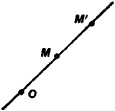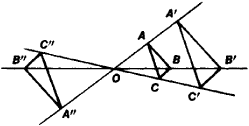# Central Similarity Homothety

The following article is from The Great Soviet Encyclopedia (1979). It might be outdated or ideologically biased.

## Central Similarity (Homothety)

in mathematics, a transformation that associates to each point M (in the plane or in space) a point M’ on OM, where O is a fixed point (Figure 1), such that the ratio OM’/OM =λ is the same for all M different from O (the ratio OM’/OM is considered positive if the points M’ and M are on the same side of O and negative otherwise). The point O is called the center and the number λ is called the coefficient of the transformation. Depending on the sign of λ. we speak of a central similarity with positive or negative similarity coefficient. A central similarity with center O and coefficient -1 is a half turn about O. A central similarity maps lines onto lines. It also preserves parallelism of lines and planes and the magnitude of (plane and dihedral) angles. If a figure F’ is the image of a figure F under a central similarity, then F and F’ are similar (Figure 2). A central similarity can be defined as an affine transformation such that the lines joining corresponding points pass through the same point (the center of the central similarity). Central similarities are used to enlarge images (slide and movie projectors).Figure 1Figure 2

The Great Soviet Encyclopedia, 3rd Edition (1970-1979). © 2010 The Gale Group, Inc. All rights reserved.
Site: Follow: Share:
Open / Close